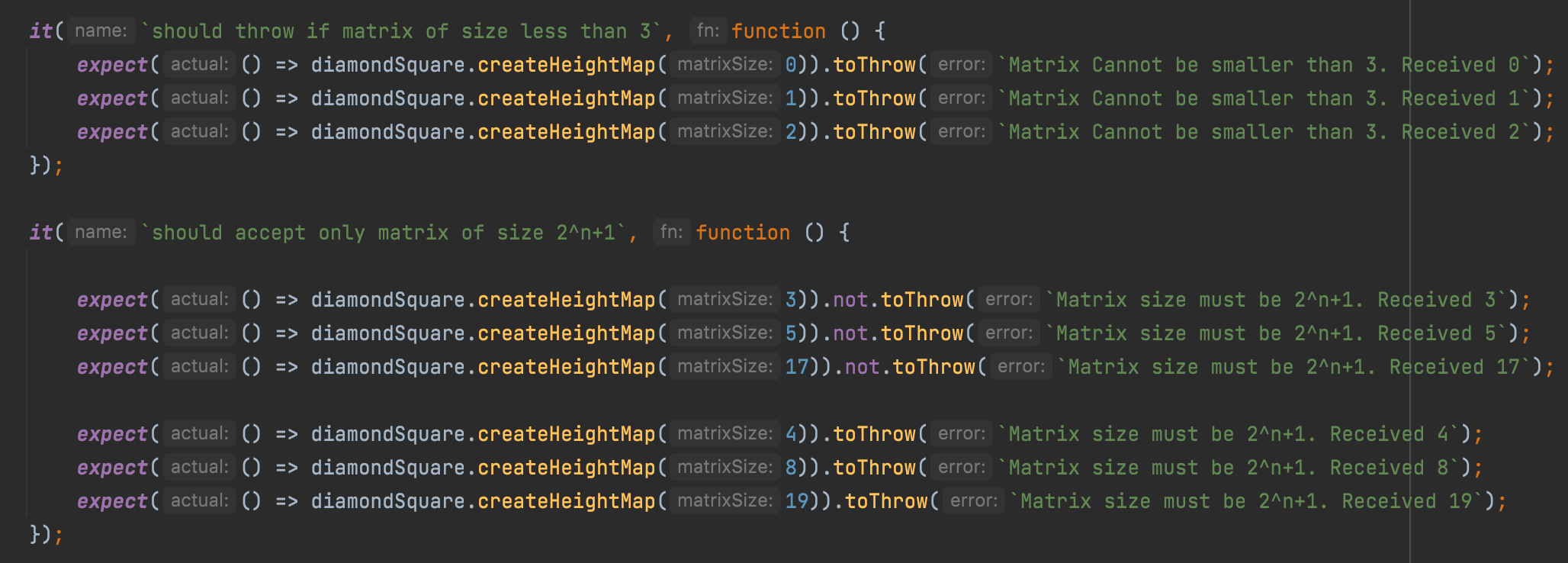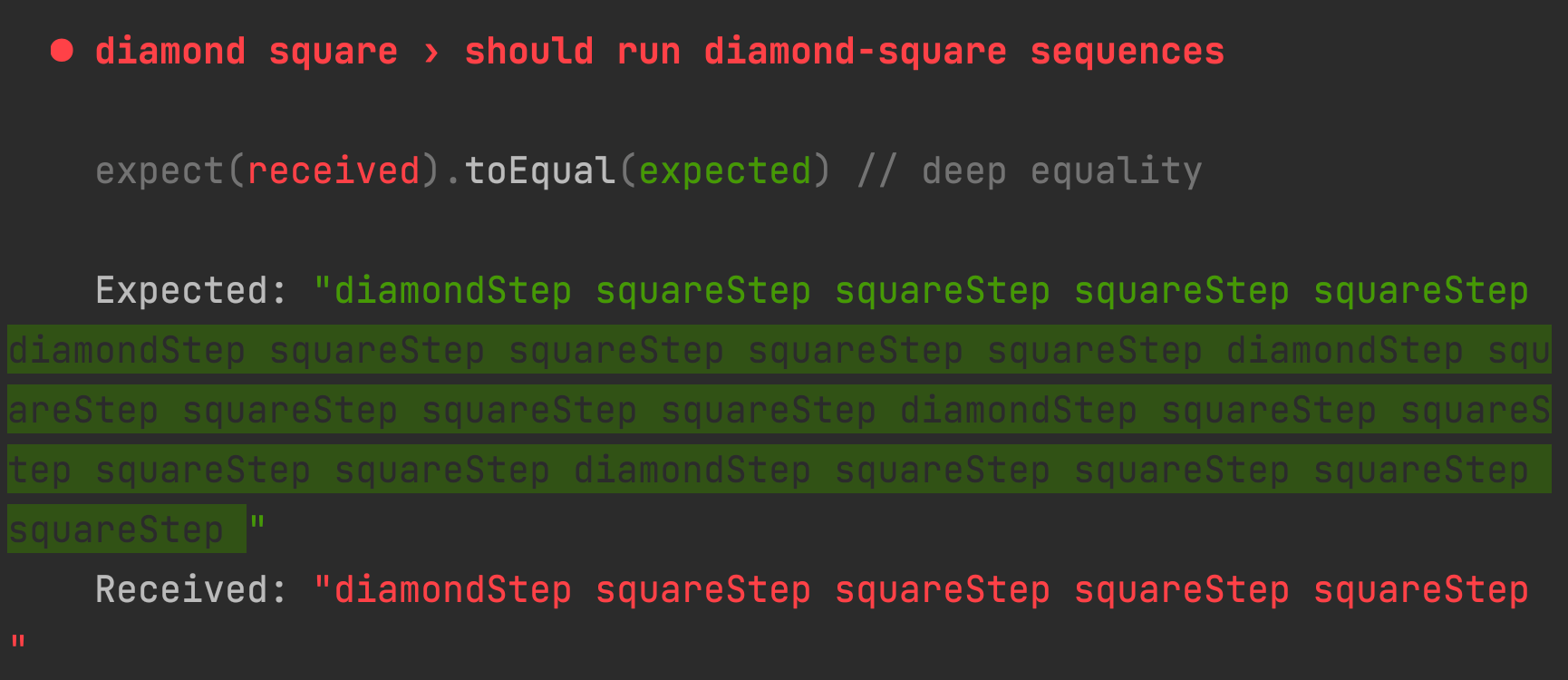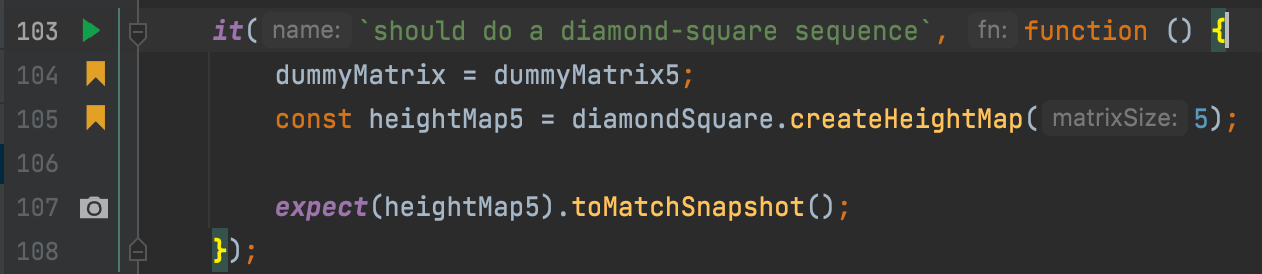# 5 lessons learned when I TDD an algorithm in JavaScript

How to develop an algorithm using TDD? In this article we will implement the Diamond-Square algorithm using TDD. This article was inspired by Uncle Bob’s blog post TDD Lesson – Terrain Generation.

Well, one of the things I did during the summer was to write an article about “How to Create Terrain and Heightmaps using the Diamond-Square Algorithm in JavaScript?“.

I just found out that Uncle Bob wrote an article about TDDing the Diamond Square algorithm (yea, I’m slow on catching up sometimes).

In the article, Uncle Bob leads us through a way to TDD an algorithm. It’s pretty nice and gave me a few insights as to how to mock and test in intervals until the algorithm emerges.

Problem is – Uncle Bob’s code is not JavaScript!!!

So I set down and reimplemented the algorithm. This time, I used the lessons learned from Uncle Bob’s article.

## What did I learn while developing an algorithm using TDD in JavaScript?

### Lesson #1: Start with the simplest test possible

In his article, Uncle Bob starts by testing input validation. That seems too simple, but I find this simplicity charming. We use to neglect input validation until we finish writing the code (if ever). Testing input validation? Most people don’t do that.

Starting with input validation is a kind of “warmup” to the real thing. I really suggest doing it. It takes a minute (or less) and is worth it. Here’s my `input validation` test:Input validation tests. This begins the TDD of the algorithm.

### Lesson #2: Mock for stability

Many algorithms have a random factor. The Diamond-Square algorithm has a random factor in the tile value calculation. On every such calculation, we calculate the mean of surrounding tiles and add some random number to make things interesting.

A random factor is not so good for tests. Unit tests need to be stable and that means that every function return should be predictable.

In some cases, using `Math.random` is enough. In these cases, we can stub `Math.random` to return a constant value for our tests.

There are other cases (like our algorithm) in which the randomness is a bit more complex. In this case, writing a function or a method to generate this randomness is a good practice.

We first create this function:

``````it(`should generate a random number that is multiplied by 2 in the power 0 to -0.1`, function () {
diamondSquare.generateRandom.mockRestore();

jest.spyOn(Math, 'random').mockReturnValue(1);
const randomFactorWithOne = diamondSquare.generateRandom(1);

jest.spyOn(Math, 'random').mockReturnValue(.1);
const randomFactorWithTenth = diamondSquare.generateRandom(1);

expect(randomFactorWithOne).toEqual(Math.pow(2, -.1));
expect(randomFactorWithTenth).toEqual(Math.pow(2, .1*-0.1));
});``````

Then we can mock it to get a constant “random” value in our test:

``jest.spyOn(diamondSquare, 'generateRandom').mockReturnValue(1);``

This magic line above mocked the `generateRandom` method and always returned the value 1.

### Lesson #3: Track your state with a string

Algorithms can become very complex. They usually iterate a lot on the data, and tracking the steps can become difficult. What Uncle Bob suggests is to stub some functions to track the steps using a string and create sequences that are easy to follow:

In the Diamond-Square algorithm we know we need to have a certain sequence of steps. We’d expect on every iteration to calculate 4 squares for every diamond. So in a 3×3 matrix we will have something like this:

``diamondStep squareStep squareStep squareStep squareStep ``

In a 5×5 matrix, we have 5 diamonds, so we will expect to have the following sequence:

``diamondStep squareStep squareStep squareStep squareStep diamondStep squareStep squareStep squareStep squareStep diamondStep squareStep squareStep squareStep squareStep diamondStep squareStep squareStep squareStep squareStep diamondStep squareStep squareStep squareStep squareStep``

So if something goes wrong, the testing framework (Jest, in my case) will be very useful in guiding me what’s missing:Missing steps in the diamond-square sequence for 5×5 matrix

I smacked my head when I read it. It reminded me “time travel” in redux and I wondered how I didn’t use this trick before.

Note that this is not always possible, because your step functions might not be be exposed from the module. The next lesson will show you a way to do it if you are OOPing.

### Lesson #4: Inheritance can be very useful for testing

If you are using OOP, you just got lucky. You can create a test class that extends your original class. This allows you to mock even without a testing framework:

While I didn’t use the full power of OOP in the actual reproduction of the algorithm, this is a nice trick that might come in handy in the future.

### Lesson #5: Avoid the “Holy Grail” test until the end

The “Holy Grail” is a term that is used to describe the end result of our algorithm or module or function.

By starting with the Holy Grail, we can really fast hit a wall. The algorithm is quite complex and the results are somewhat hard to calculate. Especially in JavaScript, where the floating numbers returned from a calculation can be quirky.

Here’s my “Holy Grail” test:The “Holy Grail” test reduced to just running the complete function and taking a snapshot. Then I just look at the snapshot and see that the calculation is correct.

## Summary

I love TDD. I believe it improves my developing experience by simplifying big tasks.

The idea is to build incrementally. Start from the simplest (the first lesson learned here) and move on to the “Holy Grail” that will emerge from the simpler tests.

As Uncle Bob himself suggests in the article – do try this at home.

For those of you who are interested, here’s the full solution:

Thanks a lot to Hod Bauer and Yair Cohen for the kind and thorough review.

Featured Photo by Paul Gilmore on Unsplash

#### Sign up to my newsletter to enjoy more content: